### 9.1.1.1 Optimizing a single objective

Before progressing to complicated decision-making models, first consider the simple case of a single decision maker that must make the best decision. This leads to a familiar optimization problem, which is formulated as follows.

Formulation 9..1 (Optimization)
1. A nonempty set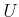called the action space. Each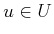is referred to as an action.
2. A function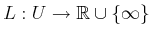called the cost function.

Compare Formulation 9.1 to Formulation 2.2. State space,, and state transition concepts are no longer needed because there is only one decision. Since there is no state space, there is also no notion of initial and goal states. A strategy simply consists of selecting the best action.

What does it mean to be the best'' action? Ifis finite, then the best action,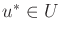is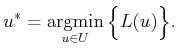(9.1)

Ifis infinite, then there are different cases. Suppose that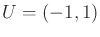and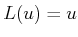. Which action produces the lowest cost? We would like to declare that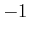is the lowest cost, but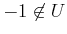. If we had instead defined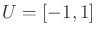, then this would work. However, ifand, then there is no action that produces minimum cost. For any action, a second one,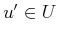, can always be chosen for which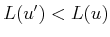. However, ifand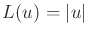, then (9.1) correctly reports that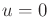is the best action. There is no problem in this case because the minimum occurs in the interior, as opposed to on the boundary of. In general it is important to be aware that an optimal value may not exist.

There are two ways to fix this frustrating behavior. One is to require thatis a closed set and is bounded (both were defined in Section 4.1). Since closed sets include their boundary, this problem will be avoided. The bounded condition prevents a problem such as optimizing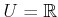, and. What is the best? Smaller and smaller values can be chosen forto produce a lower cost, even thoughis a closed set.

The alternative way to fix this problem is to define and use the notion of an infimum, denoted by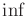. This is defined as the largest lower bound that can be placed on the cost. In the case ofand, this is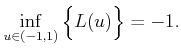(9.2)

The only difficulty is that there is no actionthat produces this cost. The infimum essentially uses the closure ofto evaluate (9.2). Ifhappened to be closed already, thenwould be included in. Unbounded problems can also be handled. The infimum for the case ofandis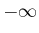.

As a general rule, if you are not sure which to use, it is safer to writein the place were you would use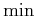. The infimum happens to yield the minimum whenever a minimum exists. In addition, it gives a reasonable answer when no minimum exists. It may look embarrassing, however, to usein cases where it is obviously not needed (i.e., in the case of a finite).

It is always possible to make an upside-down'' version of an optimization problem by multiplying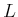by. There is no fundamental change in the result, but sometimes it is more natural to formulate a problem as one of maximization instead of minimization. This will be done, for example, in the discussion of utility theory in Section 9.5.1. In such cases, a reward function,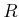, is defined instead of a cost function. The task is to select an actionthat maximizes the reward. It will be understood that a maximization problem can easily be converted into a minimization problem by setting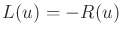for all. For maximization problems, the infimum can be replaced by the supremum,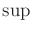, which is the least upper bound on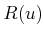over all.

For most problems in this book, the selection of an optimalin a single decision stage is straightforward; planning problems are instead complicated by many other aspects. It is important to realize, however, that optimization itself is an extremely challenging ifandare complicated. For example,may be finite but extremely large, ormay be a high-dimensional (e.g., 1000) subset of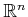. Also, the cost function may be extremely difficult or even impossible to express in a simple closed form. If the function is simple enough, then standard calculus tools based on first and second derivatives may apply. It most real-world applications, however, more sophisticated techniques are needed. Many involve a form of gradient descent and therefore only ensure that a local minimum is found. In many cases, sampling-based techniques are needed. In fact, many of the sampling ideas of Section 5.2, such as dispersion, were developed in the context of optimization. For some classes of problems, combinatorial solutions may exist. For example, linear programming involves finding the min or max of a collection of linear functions, and many combinatorial approaches exist [259,264,664,731]. This optimization problem will appear in Section 9.4.

Given the importance of sampling-based and combinatorial methods in optimization, there are interesting parallels to motion planning. Chapters 5 and 6 each followed these two philosophies, respectively. Optimal motion planning actually corresponds to an optimization problem on the space of paths, which is extremely difficult to characterize. In some special cases, as in Section 6.2.4, it is possible to find optimal solutions, but in general, such problems are extremely challenging. Calculus of variations is a general approach for addressing optimization problems over a space of paths that must satisfy differential constraints ; this will be covered in Section 13.4.1.

Steven M LaValle 2020-08-14